УДК 1; 51; 511; 519.7; 519.72; 519.76; 519.76; 539.1; 539.1.07; 539.12; 539.18; 530.145; 530.145; 681.5.07; 57; 573.3; 573.5; 572; 575.112; 61

# МАТЕМАТИЧЕСКАЯ МЕТРИКА АТОМА ВОДОРОДА КАК МОДЕЛЬНАЯ ЗАДАЧА ДЛЯ ТЕСТИРОВАНИЯ КВАНТОВОГО КОМПЬЮТЕРА И ЛОГИЧЕСКОГО ПРОГРАММИРОВАНИЯ АТОМОВ

Злобин Андрей Евгеньевич
Московский Государственный Технический Университет им. Н.Э.Баумана
кандидат технических наук, математик

Аннотация
Предложена новая модельная задача для тестирования квантового компьютера. Используется математическая метрика атома водорода. Декларируются будущие перспективы логического программирования атомов и элементарных частиц.

# MATHEMATICAL METRICS OF HYDROGEN ATOM AS MODEL TASK FOR TESTING OF QUANTUM COMPUTER AND LOGICAL PROGRAMMING OF ATOMS

Zlobin Andrei Evgenyevich
Bauman Moscow State Technical University
Ph.D., mathematician

Abstract
New model task for testing of quantum computer is suggested. Mathematical metrics of hydrogen atom is used. Future perspective of logical programming of atoms and elementary particles is declared.

Библиографическая ссылка на статью:
Злобин А.Е. Mathematical metrics of hydrogen atom as model task for testing of quantum computer and logical programming of atoms // Современные научные исследования и инновации. 2017. № 8 [Электронный ресурс]. URL: https://web.snauka.ru/issues/2017/08/84228 (дата обращения: 20.10.2021).

The author suggests new model task for testing of quantum computer. The task is based on mathematical metrics of hydrogen atom. The metrics was published by the author earlier, for example [Zlobin, 2010, 2013, 2014], and this mathematical expression seems very convenient for fast calculation with ultra high accuracy of some tasks concerning atoms.

The metrics include four constants: Ludolph number (p=3.1415…), Napier number (e=2.7182…), golden ratio (F=1.6180…) and so-called “irrational one number” (j=1.0079…). The term “irrational one number” was suggested by the author for the first time. Moreover, the constant j=1.0079… simultaneously coincides to the value of atomic mass of hydrogen with high accuracy. It is the most wonderful property of this mathematical expression. Also we can see that mathematical metrics of atom of hydrogen describes the substance, the mass, the length and the form simultaneously. Thus the expression may be used as universal system of measurements (metrics) for analysis of atoms.

There is accurate view of mathematical metrics of hydrogen atom: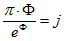certainly it mean that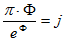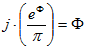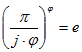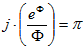where

 j=1.0079… Ф=1.6180… e= 2.7182…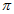=3.1415…

and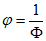It is good visible that four mathematical constantse, Ф, j are closely connected to the idea of hydrogen atom. The view of this hydrogen metrics looks like theoretical hint concerning complicated internal logical structure of the atom. Also there is probably reflection of logical properties of hydrogen atom. The perspective of logical programming of atoms and elementary particles seems visible in future. That is why the metrics as model task may be useful for testing together with logic and accuracy of quantum computer.

References
1. Zlobin, A.E. 2010, Atom of hydrogen as a metric base in organic world. Abstract of 2-nd International Congress-Partnering & Exhibition on Biotechnology and Bioenergy (EurasiaBio-2010). April 13-15, WTC, Moscow, 410-411.
2. Zlobin, A.E. 2013, Tunguska similar impacts and origin of life; arXiv:1402.1408 [physics.gen-ph]
3. Zlobin, A.E. 2014, Symmetry infringement in mathematical metrics of hydrogen atom as illustration of ideas by V.I.Vernadsky concerning origin of life and biosphere. Acta Naturae, Special issue, No. 1, p.48.